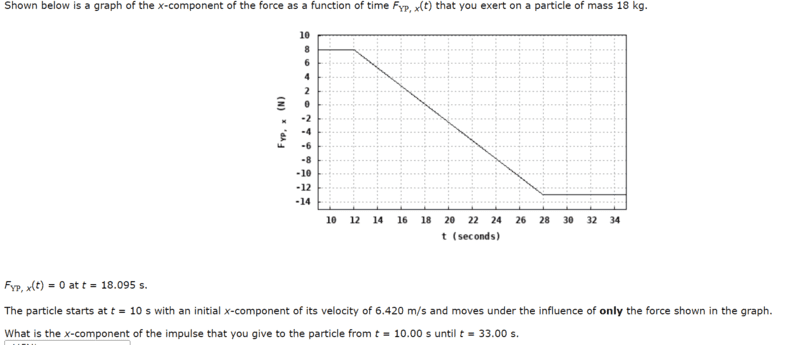# Graphical Impulse calculation from the graph of force verus time

yesmale4
Homework Statement:
Shown below is a graph of the x-component of the force as a function of time FYP, x(t) that you exert on a particle of mass 18 kg.
FYP, x(t) = 0 at t = 18.095 s.
The particle starts at t = 10 s with an initial x-component of its velocity of 6.420 m/s and moves under the influence of only the force shown in the graph.

What is the x-component of the impulse that you give to the particle from t = 10.00 s until t = 33.00 s.
Relevant Equations:
J=F*(t-t0)
area of triangle
area of rectanglehello i would like to get some help with this problem.
At first it try to calculate the impulse by the area but i found it too difficult
Then i try to solve it by the forumla J= F(t-t0), but the problem is that i don't know what F is so i try to solve it like this
F10) = 8
F(33) = -13
so
EF = 8+-13 = -5
T = 33 -10 = 23
F = 23 * -5 = -115
and it wrong so i would like to know what I am supposed to do here

Homework Helper
Gold Member
2022 Award
EF = 8+-13 = -5
What do you think you have calculated there?
How did you try to do it by graph area? Describe the steps.

yesmale4
What do you think you have calculated there?
How did you try to do it by graph area? Describe the steps.
about the first question i was thinking that because impulse formula is J=F*(t-t0) and the force isn't constant then if i calculate the change in the force and multiply it by the change of time it will be alright
about the second question i was not able to calculate the area between t = 12 to t= 28 because i don't undertand what kind of shpe it is , its not triangle so that's why i try diffrent method

Homework Helper
Gold Member
2022 Award
i was thinking that because impulse formula is J=F*(t-t0) and the force isn't constant then if i calculate the change in the force and multiply it by the change of time it will be alright
If a car drove at one speed for a while, slowed down to a different speed, then drove at that speed for a while, would you find the distance traveled by multiplying the change in speed by the time taken?
What if you were to increase the speed by 1m/s at all stages? You would calculate the same number. What if you increased the time at the higher speed and reduced the time at the lower speed by the same? Again, you would calculate the same number.
i was not able to calculate the area between t = 12 to t= 28 because i don't undertand what kind of shpe it is , its not triangle
So cut it into simpler shapes.